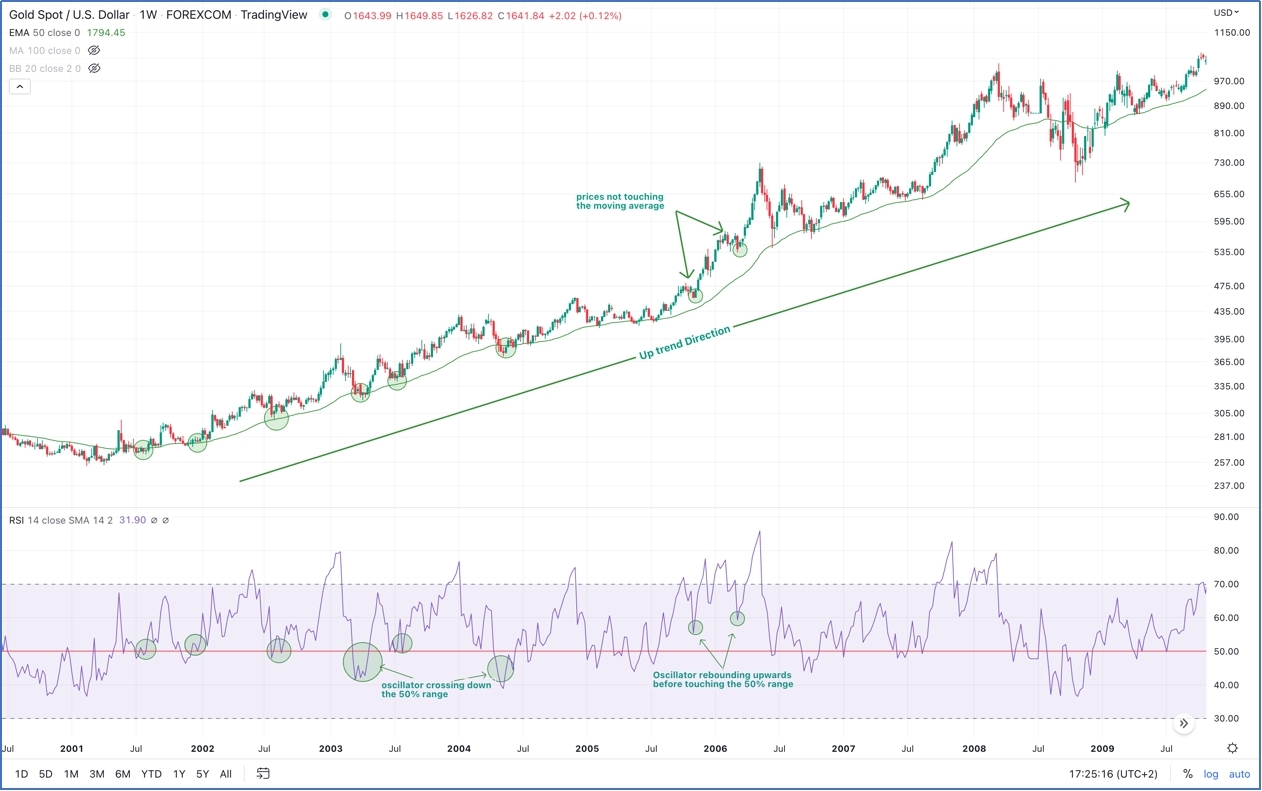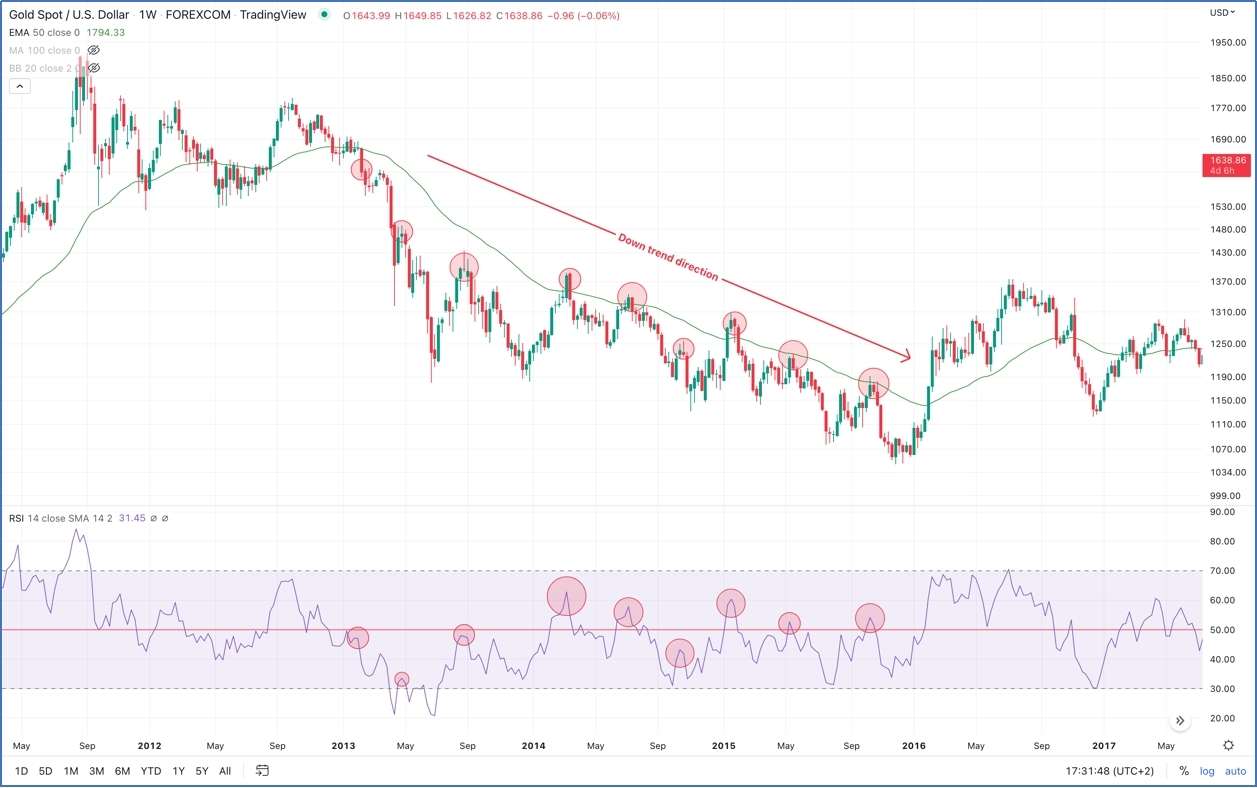# Combining Relative Strength Index With Moving Averages

### Moving Average

A moving average is a lagging technical analysis indicator of which its main function is to smooth prices thus decreasing price noise and creating constantly updated average price. That said, moving averages would identify price trends and, support and resistance levels.

Moving averages can be set for different periods. The most used periods are 10, 20, 50, 100 and 200 moving averages. The longer the moving averages, the greater the lag, the 200 days moving average will have greater lag than the 20 days moving average because it contains price averages for 200 days be it simple, weighted or an exponential moving average.

### RSI indicator

The relative strength index (RSI) is a technical momentum indicator that compares recent price gains against recent price losses, it is a momentum indicator measuring the magnitude of price movements. The RSI is a bounded indicator from zero to 100, reading above 70 usually indicates an overbought condition while readings below 30 usually indicate that the asset is in an oversold condition, that’s how it is utilized by traders. However, overbought, and oversold assets do not necessarily rebound right away meaning that it is highly recommended to get confirmation from another indicator to verify the buy/sell decision and not to rely solely on RSI signals.

The RSI calculation formula: the RSI calculates the ratio of up closes to down closes over a specific amount of time. The resulting curve is an oscillator moving between 0 and 100. The formula of the RSI is as follows

RSI= 100-(100/1+RS)

Where the RS is the average up closes of the last N days divided by the average down close of the same period.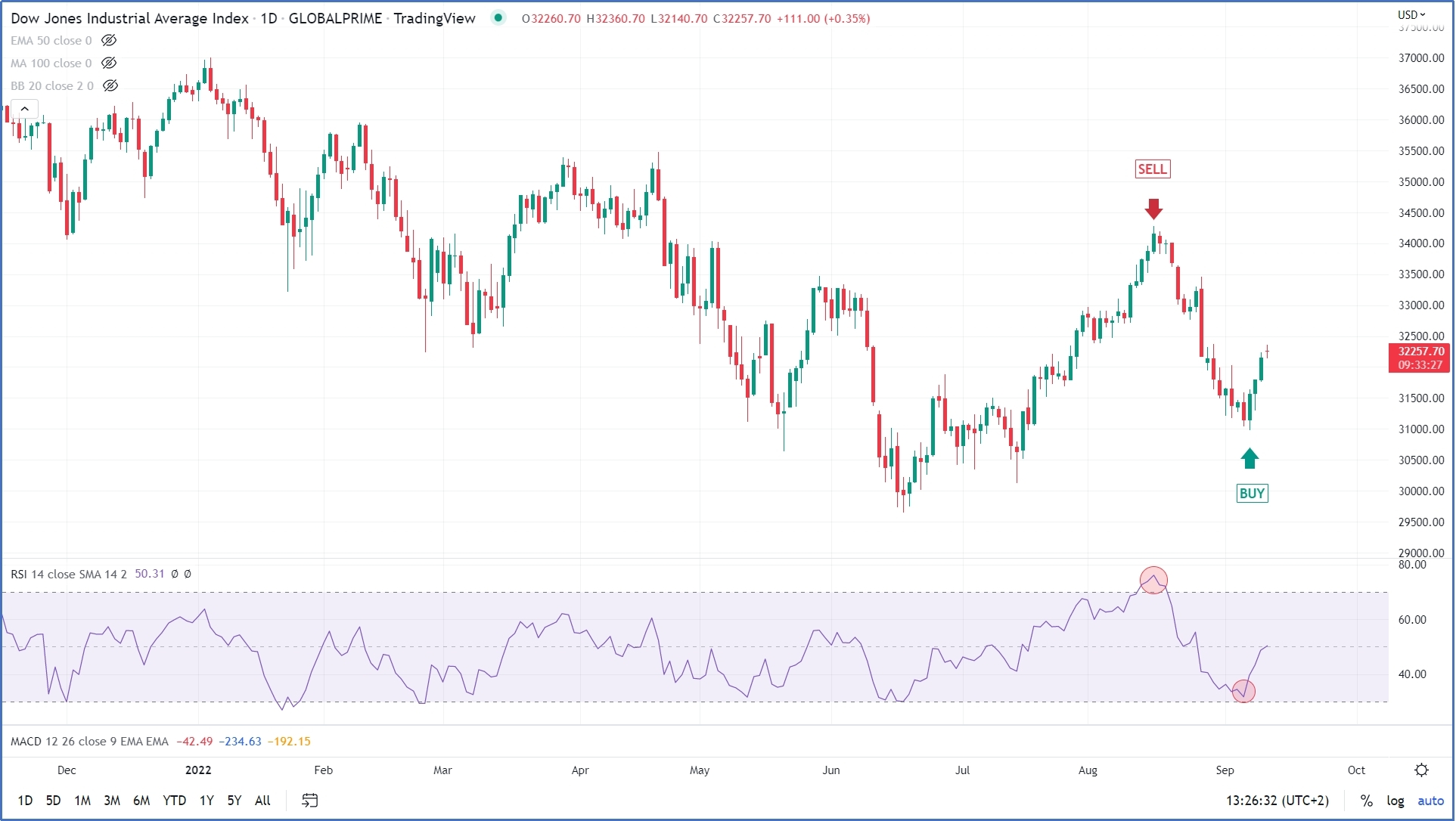1- Overbought/ over sold: the over bought is defined as when the indicator lies anywhere above the 70 levels and the oversold zone is when the indicator is anywhere below the 30 level. Accordingly, a simple strategy is to sell when the RSI crosses down the 70 levels and buy when the RSI crosses up the 30 levels.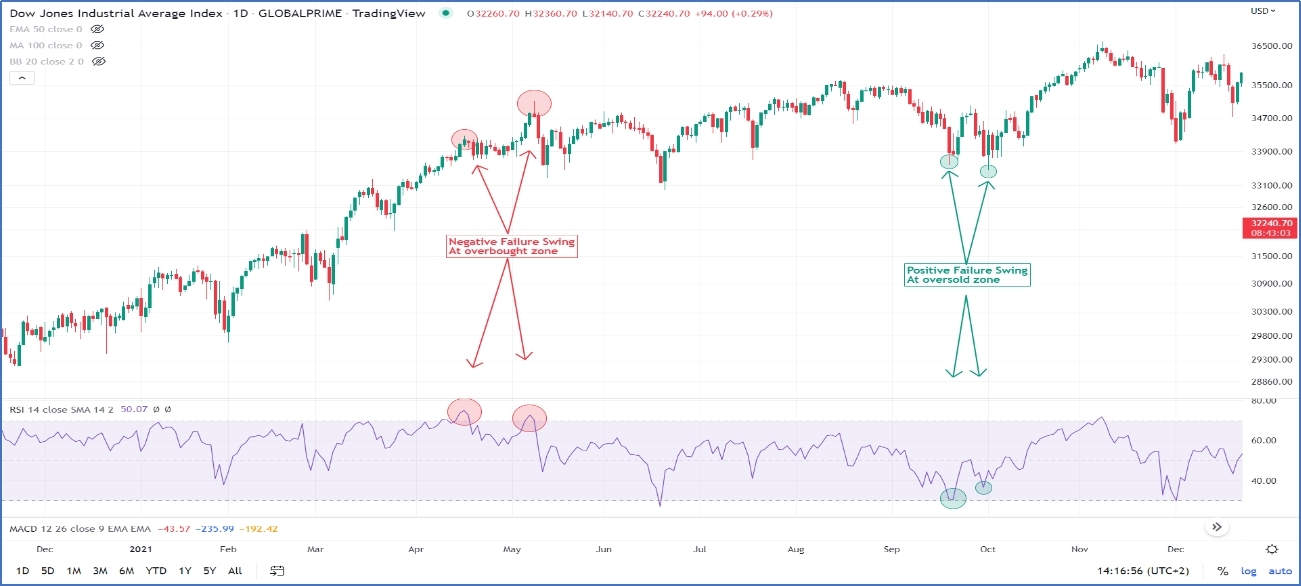2- Failure swing: a negative failure swing occurs when the RSI reaches the overbought area (over 70) declines and then rises again to a level below the first peak. The sell signal is triggered when the bottom connecting the two peaks is violated. A positive failure swing occurs when the RSI breaks the 30 to the downside, rises, and then declines to a level above the first bottom. An upside breaks of the peak connecting the two bottoms is a buy signal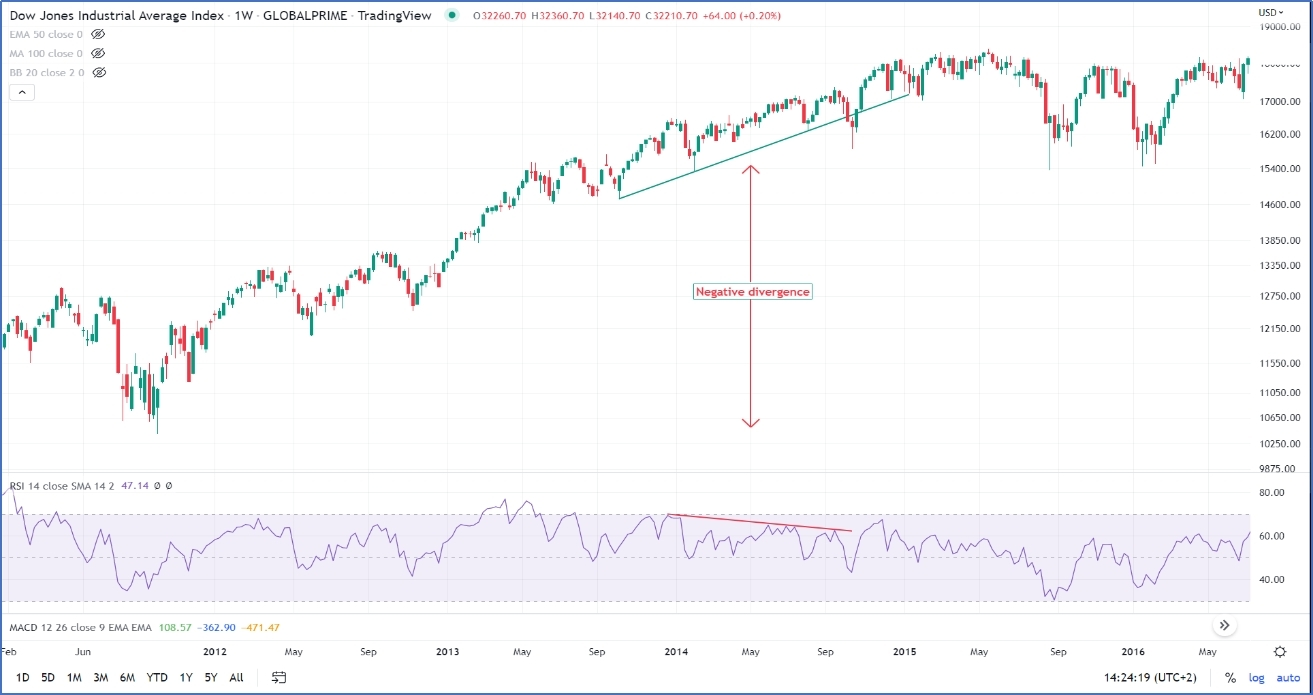3- Divergence: a positive diverge occurs when eh prices make a lower low, while RSI makes a higher low. Usually, divergences are stronger if occurred at oversold levels. A negative divergence occurs when prices make a higher high while the RSI makes lower high, negative divergences are stronger at overbought levels.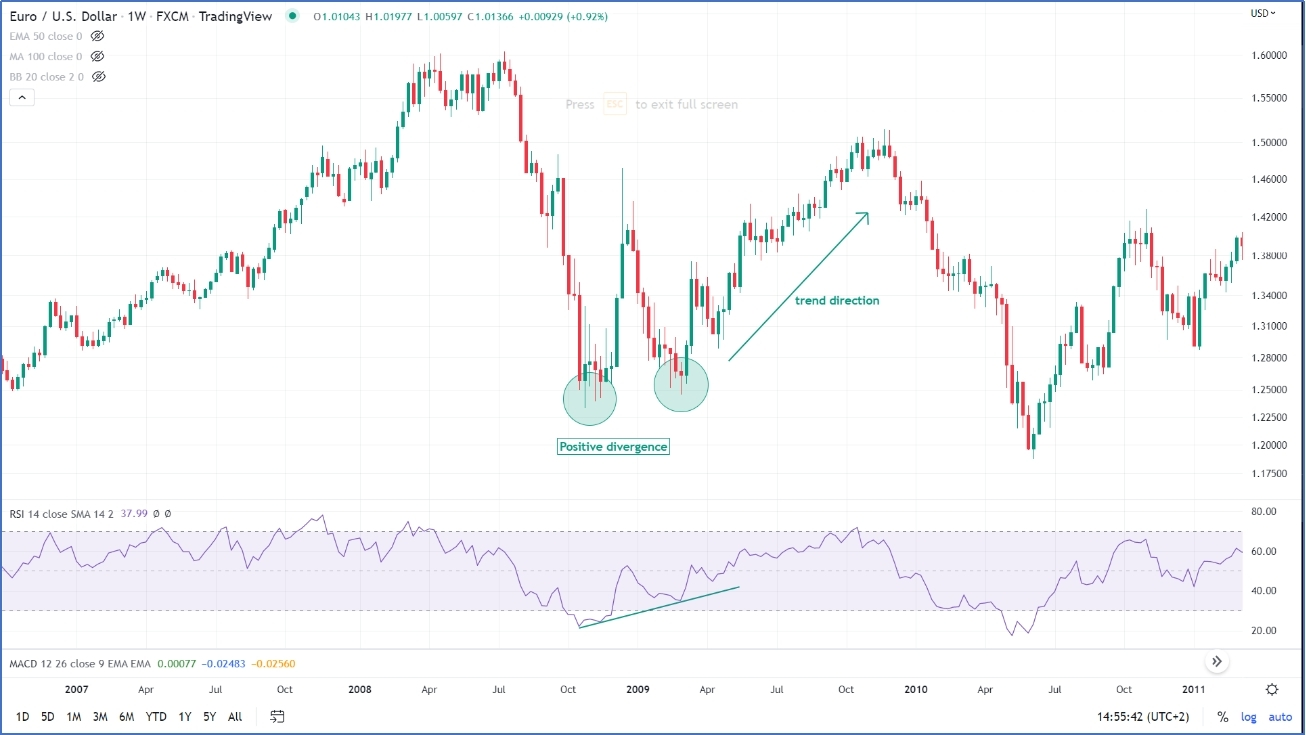### Trading the RSI with moving averages

As stated above, moving averages are lagging indicators mainly used to identify trends while the RSI is a leading momentum indicator which signals over bought and oversold status of the security by measuring the magnitude of price movements. Accordingly, it is not recommended applying this strategy in a ranging market.

Rely on the concept that moving averages act as support and resistance entry and exit levels can be identified as follows:

Price crossover: in a trending market, a trader can take a long position when the RSI crosses the 50% upward and the prices close above the moving average. Same applies for taking a short position when the RSI crosses the 50% range downward and prices close below the moving average. The drawback of this tactic is that the trader might fall in the pitfall of whipsaws or false breaks and overtrading.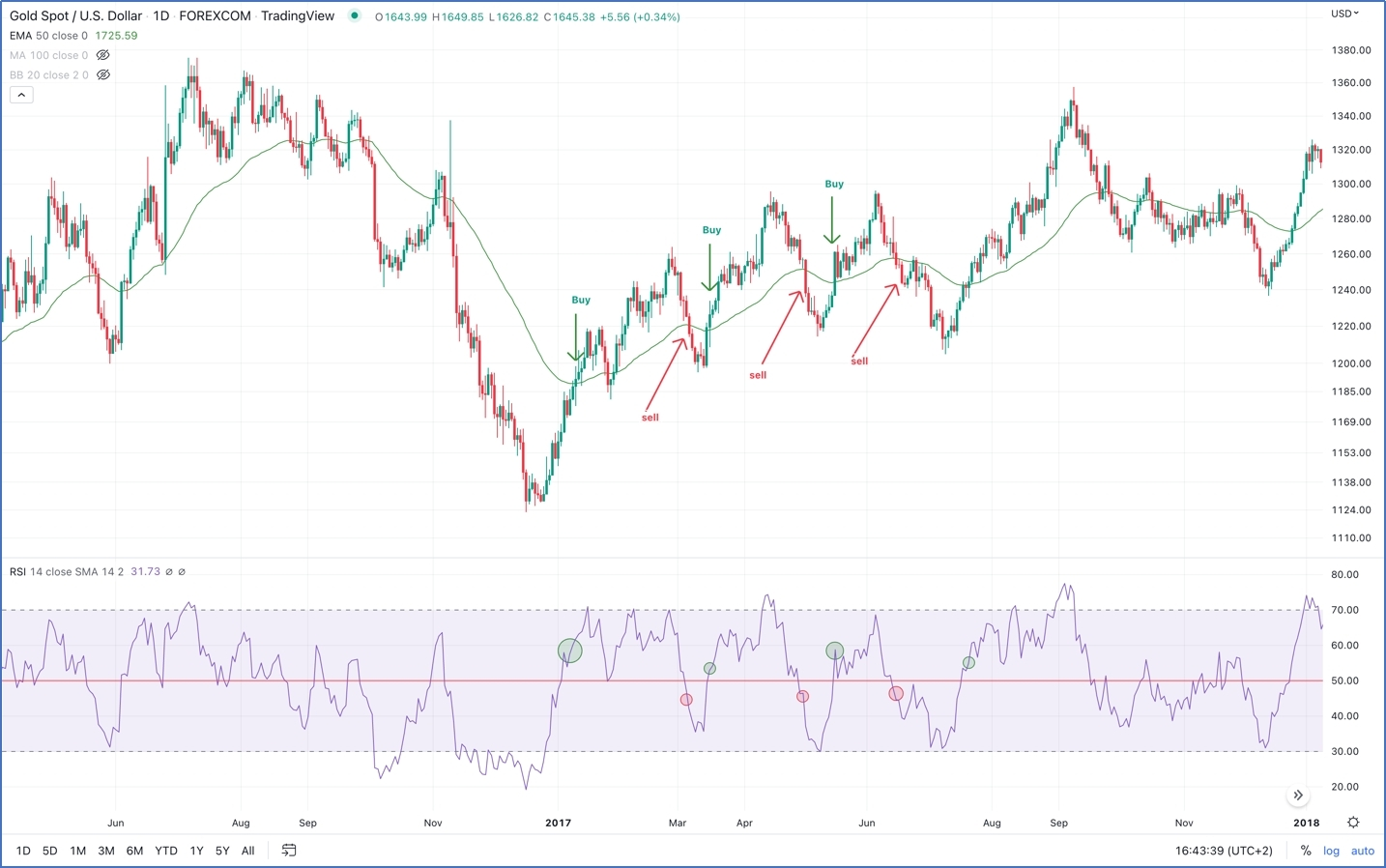Price crossover: in a trending market, a trader can take a long position when the RSI crosses the 50% upward and the prices close above the moving average. Same applies for taking a short position when the RSI crosses the 50% range downward and prices close below the moving average. The drawback of this tactic is that the trader might fall in the pitfall of whipsaws or false breaks and overtrading.